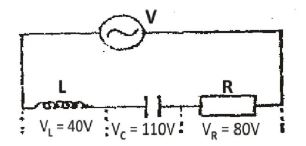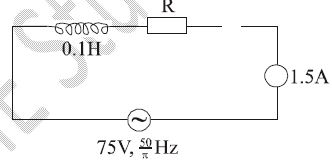Physic Tutorials

# How to solve questions on alternating current circuit for UTME

How to solve questions on alternating current circuit for UTME

Question 1

The current output form of an a.c. source is given as I = 10 sin w t. The d.c. equivalent of the current is {UTME 2012}
A. 5.0A       B. 7.1A        C. 10.0A        D. 14.1A

Solution

I = I0 sin wt

Comparing this with the equation in the question

Io = maximum current = 10 A

The dc equivalent of the current = Irms

Irms = 0.7071*I0 = 0.7071 * 10 = 7.1 A

Question 2

In an a.c. circuit, the ratio of r.m.s value to peak value of current is {UTME 2011}
A. √2    B. 2       C. 1/2         D. 1/√2

Solution

Irms = (1/√2) I0

Irms / I0 = 1/√2

Question 3

In alternating current circuit at resonance, the angle of lead or lag is {UTME 2010}

A. π/2    B. 0     C. π/3      D. π

Solution

At resonance Xl(inductive reactance) = Xc(capacitive reactance)

The angle of lead or lag = 0

B is the correct option

Question 4From the diagram above, if the potential difference across the resistor, capacitor and inductor are 80V, 110V and 40V respectively, the effective potential difference is{UTME 2009}
A. 116.3V        B. 50.0V         C. 230.0V      D. 106.3V

Solution

V^2 = Vr^2 + (Vc – Vl)^2

V = √(Vr^2 + (Vc – Vl)^2)

V = √(80^2 + (110-40)^2)

V = √6400 + 4900 = √11300

V = 106.3 v

D is the correct option

Question 4

The d.c. generator has essentially the same components as the a.c. generator except the presence of{UTME 2008}
A. slip-ring          B. carbon brushes         C. split ring         D. armature

Solution

The only difference between a.c and d.c generator is that d.c uses split ring which enables current to be maintained in only one direction while a.c generator uses slip-ring which enables the cureent to flow sinusoidally.

d.c has split ring

C is the correct option

Question 5

The instantaneous value of the induced e.m.f. as a function of time is ε = εo sinwt where εo is the peak value of the
e.m.f. The instantaneous value of the e.m.f, one quarter of the period, is{UTME 2007}

A. εo    B. εo/2       C.  εo/4       D. 0

Solution

w = 2πf

f = 1/T

w = 2π / T

one quarter of the period will be

w = 2π / (T/4) =4* (2π / T)

ε = εo/4

C is the correct option

Question 6From the diagram, the inductive reactance and the resistance R are respectively{UTME 2007}
A. 10Ω and 50Ω       B. 20Ω and 50Ω          C. 25Ω and 50Ω       D. 50Ω and 45Ω

Solution

Xl = 2πFL  = 2*π*50/π * 0.1 =10Ω

V = IZ

75 = 1.5*Z

Z = 75 /1.5 = 50Ω

Z = √R^2 + Xl^2

50 = √R^2 + 10^2

50^2 = R^2 + 100

2500 = R^2 + 100

R^2 = 2400

R = √2400 = 49.98Ω  approximately = 50Ω

A is the correct option

Question 7

A transformer is rated 240V. If the primary coil is 4000 turns and the secondary voltage 12V, determine the number of turns in the secondary coil.{UTME 2005}
A. 100                   B. 150                     C. 200                   D. 250

Solution

Vp / Vs = Np / Ns

240 / 12 = 4000 / Ns

Ns = ( 4000 * 12) / 240

Ns = 200 v

C is the correct option

Recommended: Short note on alternating current

### Bolarinwa Olajire

A lecturer, Educationist, PhD student at FUNAAB, and a Blogger.